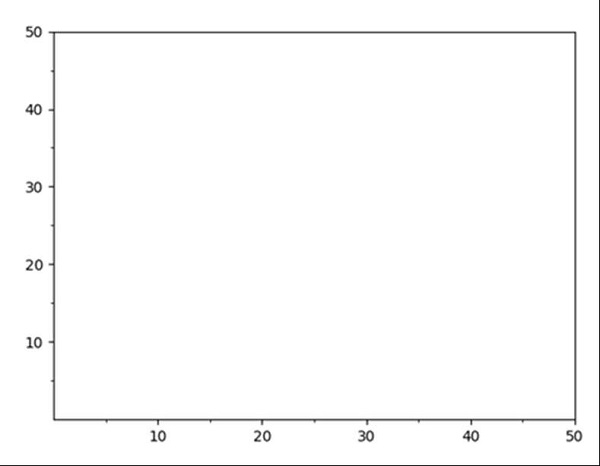# Change grid interval and specify tick labels in Matplotlib

Using plt.figure() method, we can create a figure and thereafter, we can create an axis. Using set_xticks and set_yticks, we can change the ticks format and ax.grid could help to specify the grid interval.

## Steps

• Create a new figure, or activate an existing figure, using fig = plt.figure() method.

• Add an ~.axes.Axes to the figure as part of a subplot arrangement, where nrow = 1, ncols = 1 and index = 1.

• Get or set the current tick locations and labels of the X-axis.

• Get or set the current tick locations and labels of the X-axis. With minor = True, Grid.

• Get or set the current tick locations and labels of the Y-axis.

• Get or set the current tick locations and labels of the Y-axis. With minor = True, Grid.

• Lay out a grid in current line style, using grid() method.

• To show the figure we can use the plt.show() method.

## Example

import matplotlib.pyplot as plt

fig = plt.figure()
major_tick = [10, 20, 30, 40, 50]
minor_tick = [5, 15, 25, 35, 45]
ax.set_xticks(major_tick) # Grid
ax.set_xticks(minor_tick, minor=True)
ax.set_yticks(major_tick) # Grid
ax.set_yticks(minor_tick, minor=True)

ax.grid(which='both')

ax.grid(which='minor', alpha=1)
ax.grid(which='major', alpha=2)

plt.show()

## Output